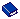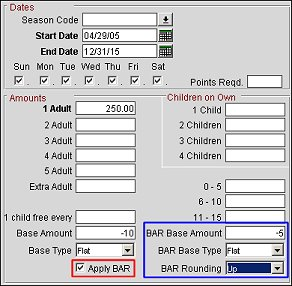BAR Applied to Rate Detail (with Advanced Dynamic Base Rates)

It is possible to configure an Advanced Dynamic Base Rate such that the amount calculated by the advanced dynamic base rate's rate detail for that date, room type and number of persons is based on the comparable BAR. (For example, the advanced dynamic base rate detail might be 10% off the BAR for that date, room type and number of persons). Moreover, the advanced dynamic base rate's calculated rate detail amount can be compared to the amount calculated using the advanced dynamic base rate's base rate to determine the amount automatically returned by an availability search.

To use the BAR Applied to Rate Detail feature, the Rates>BAR Applied to Rate Detail application parameter must be set to Y. (This parameter is available when the Rates>Best Available Rates and Rates>Advanced Dynamic Base Rates application functions are set to Y, and Rates>Best Available Rate Type application setting is set to BEST BAR BY DAY.)

When a Base Rate has been selected in the More tab's Advanced Dynamic Base Rates section, the Base Amount and Base Type fields are available on the Rate Details tab. If the BAR Applied to Rate Detail parameter is active, the Apply BAR check box is also available on the Rate Details tab under the Base Type field.Select this check box to indicate which rate details will be BAR based.

Note: The BAR can be used to calculate some rate details, the advanced dynamic base rate's base can be used for other rate details, and a defined per-person rate amount can be used for still other rate details.

The Compare with Rate Detail check box in the Advanced Dynamic Base Rate section on the More tab directs OPERA to compare theAs with "standard" Advanced Dynamic Base Rates, the Base Rate is the rate code on which the rate details for the current rate code are to be based. Rounding also behaves the same as for standard Advanced Dynamic Base Rates. However, when the Compare with Rate Detail check box is selected, the rate amount calculated on the rate detail using the Advanced Dynamic Base Rate's base rate code is compared to the amount calculated using the BAR rate (for that date and the same number of persons and room type); an availability search will return the lower of the two rates.

Example 1
Assume the BAR for 12/01/10, room type DLX, 2 persons is rate code BFST (amount = 175.00). Also assume that Advanced Dynamic Base Rate rate code ADVDYNAMIC is configured with a CORP base rate (for 12/01/10, all room types, 2 persons, the CORP rate = 150.00). Compare with Rate Detail is selected for ADVDYNAMIC.
On the ADVDYNAMIC rate detail, a -10 Base Amount is coupled with a Flat Base Type for 12/01/10 - 12/31/10. Apply BAR is selected.
The reservation agent performs a rate query on ADVDYNAMIC for 12/01/10, 2 persons, DLX room type. The rate amount returned is 140.00 because the BFST BAR rate -10 = 165.00, which is greater than the advanced dynamic base rate 's CORP base rate -10 (150.00 -10 = 140.00).
Example 2
Assume the BAR for 12/01/10, room type DLX, 2 persons is rate code BFST (amount = 175.00). Also assume that Advanced Dynamic Base Rate rate code ADVDYNAMIC is configured with CORP base rate (for 12/01/10, all room types, 2 persons, the CORP rate = 150.00). Compare with Rate Detail is NOT selected for ADVDYNAMIC.
On the ADVDYNAMIC rate detail, a -10 Base Amount is coupled with a Flat Base Type for 12/01/10 - 12/31/10. Apply BAR is selected.
The reservation agent performs a rate query on ADVDYNAMIC for 12/01/10, 2 persons, DLX room type. The rate amount returned is 165.00 because the BFST BAR rate -10 = 165.00 and a comparison with a calculation using the advanced dynamic base rate 's CORP base rate was not made.

When the Rates>BAR Applied to Rate Detail application parameter is set to Y, the Rates>BAR Details Display Option application parameter is available. When this parameter is set to Y and the Apply BAR check box is selected, the BAR Base Amount, BAR Base Type, and Rounding fields are displayed on the Rate Details screen (as seen outlined with the Blue rectangle above). When these fields are populated, these details will apply just as they would on the Rate More tab, but these will only apply to the Start/End Date Range that is specified.

Note: If values are defined on both the Rate Detail and Rate More tabs for BAR Base, then the BAR Base values defined on the Rate Detail tab will be used and the values on the Rate More tab are ignored.

Example 1
Assume the BAR for 12/01/12, room type DLX, 1 person is rate code GPLAIN (amount = \$100.00). Also assume rate code GBAR BAR rate for room type DLX (amount = 90.00). Also assume that Advanced Dynamic Base Rate rate code GBARADV is configured with GPLAIN rate code.
On the GBARADV rate detail, +10 Base Amount with Flat Base Type, Apply BAR checked, and +5 BAR Base Amount with Flat BAR Base Type.
The reservation agent performs a rate query on GBARADV for 12/01/12, 1 person, DLX room type. The rate amount returned is 95.00 because the Advanced Base Rate of 110.00 (100.00+10) is higher than the Best Available Rate 95.00 (90.00+5).